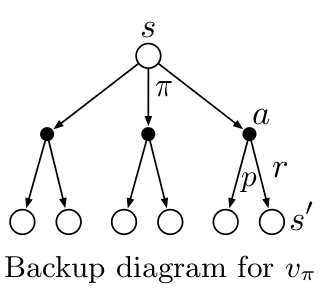# Policy Evaluation

## Policy Evalutaion

Given a MDP and a policy $\pi$, how can we measure the goodness of this policy? Policy evaluation is to find the true value function for the given policy. It uses the Bellman equation. Obtaining a true value function with the current policy is done with one step backup.

Backup means that the current values are calculated using the next values.

- One step backup vs. multi-step backup
- Full-width backup vs. sample backup (through sampling)


## Policy Evaluation for MDP

The Bellman equations are simplified as follows:

(assumes in discrete state space)

\begin{aligned} V^{\pi}(s) &= R^{\pi}(s) + \gamma E[V^{\pi}(s_{t+1}) \vert s_t = s, a_t = \pi(a \vert s)] \\ &= R^{\pi}(s) + \gamma \sum_{s' \in S} P(s' \vert s, \pi(a \vert s)) V^{\pi}(s') \end{aligned} \begin{aligned} Q^{\pi}(s, a) &= R(s, a) + \gamma E_{\pi}[Q^{\pi}(s_{t+1}, a_{t+1}) \vert s_t = s, a_t = a] \\ &= R(s, a) + \gamma \sum_{s' \in S} P(s' \vert s, a)) V^{\pi}(s') \end{aligned}

## Dynamic Programming

Dynamic programming is developed by Richard Bellman in the 1950s. It is kind of mathematical optimization algorithm, and it simplify a complicated problem by breaking it down into a sequence of simpler sub-problems in a recursive equation.

• Examples
• Dijkstra’s algorithm for the shortest path problem
• The Bellman-Ford algorithm for finding the shortest distance in a graph
• The Viterbi algorithm for hidden markov model (HMM)

## Dynamic Programming for solving finite MDP with a policy

• Iterative Policy Evaluation
• Initialize values $V_0(s) = 0$ for all states $s$
• Repeat until convergence
• For each state $s \in S$, $V_{k}^{\pi}(s) = R(s) + \gamma \sum_{s' \in S} P(s' \vert s, \pi(a \vert s)) V_{k-1}^{\pi}(s')$

This is called a Bellman (synchronous) backup for a policy $\pi$. And this recursive algorithm converges when $\gamma < 1 \text{ or } T_{ss’}^{\pi} < 1$ for all $s, s’ \in S$.

## Backup Diagram for policy Evaluation

$V^{\pi}(s) = R^{\pi}(s) + \gamma \sum_{s' \in S} P(s' \vert s, a) V^{\pi}(s')$